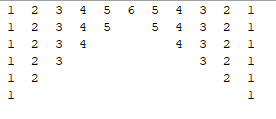WAP to Print the Double Pyramid Pattern

reena
Moderator
24 Points
11 Posts

Introduction

Following is the Double Pyramid Pattern:It's a nuber pattern. Following is the program that will print the above pattern.

Program

``package com.java;public class Pattrn {    public static void main(String[] args) {                int rows = 6;        int[] diff = {0,1,3,5,7,9,11};        int blank = 0;        int b = 0;        int temp;                for(int i = rows; i >= 1; --i){                        blank = diff[b++];            if(blank == 0)                temp = i-1;            else                temp = i;            for(int j = 1; j <= i; j++){                                System.out.print(" "+ j + " ");            }                    for(int s = 0; s < blank; s++){                System.out.print("   ");            }                        for(int k = temp; k >= 1; --k){                System.out.print(" " + k + " ");            }            System.out.println();                    }    }}``